Home MonkeyNotes Printable Notes Digital Library Study Guides Study Smart Parents Tips College Planning Test Prep Fun Zone Help / FAQ How to Cite New Title Request

Example 67

If x = a sec q , y = a tan q , Find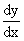at q =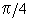Solution: x = a sec q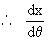= a sec q tan q

and y = a tan q= a sec2 q then,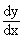=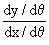=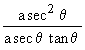=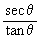==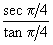=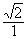=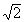Example 68

If x = a ( q - sin q ) and y = a ( 1 - cos q ). Show thatYour browser does not support the IFRAME tag.

Solution : x = a ( q - sin q )= a ( 1 - cos q )

=and y = a (1 - cos q)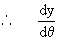= a (sin q)

=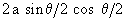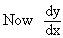===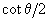Index

4. 1 Derivability At A Point
4. 2 Derivability In An Interval
4. 3 Derivability And Continuity Of A Function At A Point
4. 4 Some Counter Examples

4. 5 Interpretation Of Derivatives
4. 6 Theorems On Derivatives (differentiation Rules)
4. 7 Derivatives Of Standard Functions
4. 8 Derivative Of A Composite Function
4. 9 Differentiation Of Implicit Functions

4.10 Derivative Of An Inverse Function
4.11 Derivatives Of Inverse Trigonometric Functions
4.12 Derivatives Of Exponential & Logarithmic Functions
4.13 Logarithmic Differentiation
4.14 Derivatives Of Functions In Parametric Form
4.15 Higher order Derivatives

Chapter 5All Contents Copyright © All rights reserved.
Further Distribution Is Strictly Prohibited.

1209374 PinkMonkey users are on the site and studying right now.